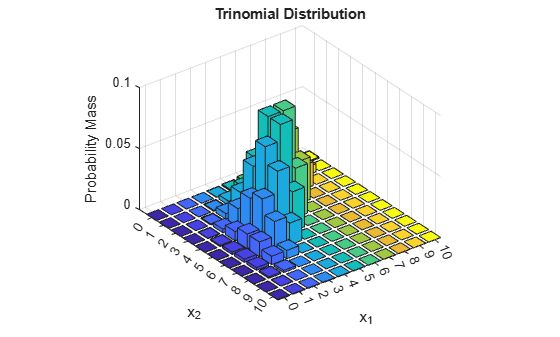# mnpdf

Multinomial probability density function

## Syntax

```Y = mnpdf(X,PROB) ```

## Description

`Y = mnpdf(X,PROB)` returns the pdf for the multinomial distribution with probabilities `PROB`, evaluated at each row of `X`. `X` and `PROB` are m-by-k matrices or 1-by-k vectors, where k is the number of multinomial bins or categories. Each row of `PROB` must sum to one, and the sample sizes for each observation (rows of `X`) are given by the row sums `sum(X,2)`. `Y` is an m-by-1 vector, and `mnpdf` computes each row of `Y` using the corresponding rows of the inputs, or replicates them if needed.

## Examples

collapse all

Compute the pdf of a multinomial distribution with a sample size of `n = 10`. The probabilities are `p = 1/2` for outcome 1, `p = 1/3` for outcome 2, and `p = 1/6` for outcome 3.

```p = [1/2 1/3 1/6]; n = 10; x1 = 0:n; x2 = 0:n; [X1,X2] = meshgrid(x1,x2); X3 = n-(X1+X2);```

Compute the pdf of the distribution.

`Y = mnpdf([X1(:),X2(:),X3(:)],repmat(p,(n+1)^2,1));`

Plot the pdf on a 3-dimensional figure.

```Y = reshape(Y,n+1,n+1); bar3(Y) h = gca; h.XTickLabel = [0:n]; h.YTickLabel = [0:n]; xlabel('x_1') ylabel('x_2') zlabel('Probability Mass') title('Trinomial Distribution')```Note that the visualization does not show `x3`, which is determined by the constraint `x1 + x2 + x3 = n`.

## Version History

Introduced in R2006b